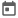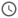# Loops in system verilog##### 16 Jun 2022##### 5 mins

Loops in system Verilog is pretty much the same as we have in Verilog. In Verilog we saw that inside loop we cannot go out of the loop using break and continue as there were no break and continue function available in Verilog. In System Verilog we have break and continue keywords and thus can be used to exit from loop. Apart from this System Verilog adds foreach loop, do-while loop. Let’s see more about loops in this article.

### What are loops?

Loops are statements which helps to perform repetitive tasks by writing it once. In testbench loops are very important as we must perform lots of repetitive task such as sampling a signal after certain duration. So, this sampling should happen repeatedly until the simulation ends.

Loops are of 2 types:

1. Finite loop – this type of loop will repeat for certain number until some conditions are not met. Once the condition evaluates to be false it will move out of the loop and execute the statements present after loop.
2. Infinite loop – this type of loop basically run for infinite iterations or until the simulation ends. In this type of loops either there is no condition evaluation, or the condition evaluates to true every time. Infinite loops can be exited with the help of break statement if required. Infinite loops should be used with great care because using it in a wrong way can lead to unexpected results or also hang the simulation.

#### Some loop terminologies

There are some important terminologies related to loops which we will be using constantly in the rest of the articles. These are briefly discussed in this section.

1. Loop declaration – This is basically where the loop is declared. This part of the loop will change for different looping construct.
2. Loop variable – This is the variable which is used to keep track of the iterations. Basically, this variable will be updated in the end of each iteration and then checked whether the updated value of the variable satisfies a condition or not. If condition is not satisfied, the loop breaks.
3. Loop body – These are the statements which will be executed in different iterations. This is basically based on logic requirements and thus independent of looping construct we are using.

System Verilog added new loop construct such as foreach loop, do-while loop and enhanced the for loop. In this section we will discuss the addition in loop construct in System Verilog. For Verilog loop constructs click here.

### For Loop

This is a finite loop in which the loop variable is checked for a certain condition before executing the loop. Most often the loop variable initialization, condition checking and loop variable updation are done on the same line.

#### Syntax

``````for ( initialization; expression; step )
<statement_or_null>``````

#### Enchancement in for loop

In Verilog, the loop variable must be declared before the loop. This can cause issues if loops using same control variable is declared in two or more procedural blocks and running simultaneously.
In System Verilog, we can declare the loop variable within the for loop. Declaring a loop variable within a for loop creates a local variable whose scope is limited to the for loop. Thus, any other loop having same loop variable cannot mutate each other.

``````module foo;
initial begin
for (int a = 0; a <= 45; a++) // Loop variable declared inside loop
...
end
initial begin
loop2: for (int a = 85; a >= 0; a--) // Same loop variable used
...
end
endmodule``````

In Verilog, another limitation is that we can only have a single initialization statement and a single step assignment. In System Verilog, we can have multiple initialization statement and multiple step assignment separated by comma `,`.

``````module foo;
initial begin
// In this for loop multiple variables are initialized
// also all the variables can be used in the loop expression
for (int cnt = 0, int i = 0; i * cnt < 250; i++ )
...
end
endmodule``````

### Do while loop

This loop is new to System Verilog and was not present in Verilog. This loop is same as while loop, but it is guaranteed that the loop will run for at least 1 iteration before coming out of loop. This is because in do-while the condition is mentioned in the end of the loop construct and thus, the condition is evaluated in the end of the iteration.

#### Syntax

``do <statement_or_null> while (expression);``

### Foreach loop

Foreach is a special type of loop which is used to iterate within different elements of arrays or queue. In this looping construct, we pass the array as the argument and the loop variable along with it inside the square bracket. The number of loop variable will depend upon the dimension of array. Each loop variable will correspond to one dimension.

Looking into the syntax and example will help understand easily.

#### Syntax

``foreach (array_identifier [loop_variables]) <statement>``

#### Mapping of Loop Variable to array index

The loop variable is mapped to array indices using the dimension cardinality. Dimension cardinality is basically the number of variables in each dimension.

``````// Unpacked array
//     1  2  3  -> Dimension Number
int A ;
loop1: foreach( A[i, j, k] ) ...

// Mixed array
//    3    4       1   2 -> Dimension Number
bit [5:0][2:1] B [4:0];
loop2: foreach( B[q, r, , s] ) ...``````

In the above code, forever loop in `loop1` block iterates through the array `A`. Now, for this loop, `i` will iterate from 0 to 4, `j` will iterate from 0 to 6 and `k` will iterate from 0 to 7.

For forever loop in `loop2` block, `q` will iterate from 4 to 0, `r` will iterate from 0 to 1 and `s` will iterate from 2 to 1. Notice that the 3rd loop variable is not provided. This means that the 3rd dimension will be skipped from iteration.

#### Example

``````module foreach_loop;
bit [5:0][2:1] B [4:0];
initial begin
foreach (B[q, r, , s]) begin
\$display("q = %0d, r = %0d, s = %0d", q, r, s);
end
end
endmodule``````
##### Output
``````# q = 4, r = 0, s = 2
# q = 4, r = 0, s = 1
# q = 4, r = 1, s = 2
# q = 4, r = 1, s = 1
# q = 3, r = 0, s = 2
# q = 3, r = 0, s = 1
# q = 3, r = 1, s = 2
# q = 3, r = 1, s = 1
# q = 2, r = 0, s = 2
# q = 2, r = 0, s = 1
# q = 2, r = 1, s = 2
# q = 2, r = 1, s = 1
# q = 1, r = 0, s = 2
# q = 1, r = 0, s = 1
# q = 1, r = 1, s = 2
# q = 1, r = 1, s = 1
# q = 0, r = 0, s = 2
# q = 0, r = 0, s = 1
# q = 0, r = 1, s = 2
# q = 0, r = 1, s = 1``````
Try this code in EDA Playground